# High School Chemistry : Using Significant Figures

## Example Questions

← Previous 1 3

### Example Question #1 : Using Significant Figures

How many significant figures are in the given number?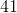Explanation:

Let's look at the rules of significant figures:

I. All nonzero digits are significant.

II. Any zero found anywhere between nonzero digits is significant.

III. Any zero to the right of the decimal point AND after a nonzero digit is significant.

IV. Any zero to the left of the decimal point AND after a nonzero digit is significant. Any zero found after a nonzero digit with no decimal point indication is NOT significant.

If we apply rule I, the answer should be two significant figures sinceis a two-digit number.

### Example Question #2 : Using Significant Figures

How many significant figures are in the given number?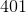Explanation:

Let's look at the rules of significant figures:

I. All nonzero digits are significant.

II. Any zero found anywhere between nonzero digits is significant.

III. Any zero to the right of the decimal point AND after a nonzero digit is significant.

IV. Any zero to the left of the decimal point AND after a nonzero digit is significant. Any zero found after a nonzero digit with no decimal point indication is NOT significant.

Let's look at rule I first. In, there are two nonzero digits. According to rule II, the zero is significant as well.has three significant figures.

### Example Question #3 : Using Significant Figures

How many significant figures are in the given number?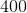Explanation:

Let's look at the rules of significant figures:

I. All nonzero digits are significant.

II. Any zero found anywhere between nonzero digits is significant.

III. Any zero to the right of the decimal point AND after a nonzero digit is significant.

IV. Any zero to the left of the decimal point AND after a nonzero digit is significant. Any zero found after a nonzero digit with no decimal point indication is NOT significant.

Let's look at rule I.only has one nonzero digit, and two zeroes. So far, there's one significant digit. There is no decimal point present, so according to rule IV the zeroes are insignificant.only has one significant figure.

### Example Question #4 : Using Significant Figures

How many significant figures are in the given number?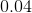Explanation:

Let's look at the rules of significant figures:

I. All nonzero digits are significant.

II. Any zero found anywhere between nonzero digits is significant.

III. Any zero to the right of the decimal point AND after a nonzero digit is significant.

IV. Any zero to the left of the decimal point AND after a nonzero digit is significant. Any zero found after a nonzero digit with no decimal point indication is NOT significant.

Let's look at rule I. In, there is only one nonzero digit and two zeroes present. That gives us one significant figure. Now, looking at rule III, the zero is to the right of the decimal but there is no nonzero digit to the left of it, making the leading zero insignificant. Finally, in rule IV, the zero after the decimal point is before the nonzero digit making and is also insignificant.contains only one significant figure.

### Example Question #1 : Using Significant Figures

How many significant figures are in the given number?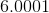Explanation:

Let's look at the rules of significant figures:

I. All nonzero digits are significant.

II. Any zero found anywhere between nonzero digits is significant.

III. Any zero to the right of the decimal point AND after a nonzero digit is significant.

IV. Any zero to the left of the decimal point AND after a nonzero digit is significant. Any zero found after a nonzero digit with no decimal point indication is NOT significant.

According to rule I, the two nonzero digits will be significant. The zeroes can be classified under rule II, as they all fall between two nonzero digits (the presence of the decimal here is negligible). The result is five sigificant figures in the given number,.

### Example Question #1 : Using Significant Figures

How many significant figures are in the given number?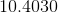Explanation:

Let's look at the rules of significant figures:

I. All nonzero digits are significant.

II. Any zero found anywhere between nonzero digits is significant.

III. Any zero to the right of the decimal point AND after a nonzero digit is significant.

IV. Any zero to the left of the decimal point AND after a nonzero digit is significant. Any zero found after a nonzero digit with no decimal point indication is NOT significant.

Rule I gives us three significant figures, as there are three nonzero digits. Next, there are two zeroes positioned between two nonzero digits; these are both significant according to rule II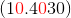. The zero to the right of the three obeys rule III and must be significant. All six of the digits inare significant.

### Example Question #3 : Using Significant Figures

How many significant figures are in the given number?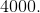Explanation:

Let's look at the rules of significant figures:

I. All nonzero digits are significant.

II. Any zero found anywhere between nonzero digits is significant.

III. Any zero to the right of the decimal point AND after a nonzero digit is significant.

IV. Any zero to the left of the decimal point AND after a nonzero digit is significant. Any zero found after a nonzero digit with no decimal point indication is NOT significant.

Rule I gives us only one nonzero significant figure. However, rule IV tells us that the zeroes are significant because there is a decimal present and the zeroes are following a nonzero digit. Therefore,has four significant figures.

### Example Question #1 : Using Significant Figures

Solve the expression using the correct number of significant figures.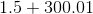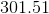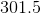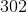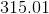Explanation:

When adding or subtracting, the final answer will have the same number of decimal places as the term with the fewest decimal places in the calculation. Always complete the calculation without rounding first to avoid errors.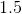has one decimal place.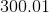has two decimal places.

Our final answer will have one decimal place.

Solve the expression and round: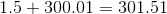Rounding to one decimal place gives us.

### Example Question #1 : Using Significant Figures

Solve the expression using the correct number of significant figures.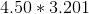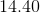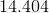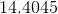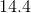Explanation:

When multiplying or dividing, the final answer will have the same number of significant figures as the term with the fewest significant figures in the calculation. Always complete the calculation without rounding first to avoid errors.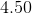has three significant figures.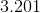has four significant figures.

Our final answer will have three significant figures.

Solve the expression and round: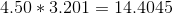Rounding to three significant figures gives us.

### Example Question #51 : High School Chemistry

Solve the expression using the correct number of significant figures.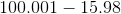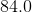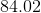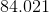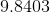Explanation:

When adding or subtracting, the final answer will have the same number of decimal places as the term with the fewest decimal places in the calculation. Always complete the calculation without rounding first to avoid errors.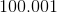has three decimal places.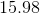has two decimal places.

Our final answer will have two decimal places.

Solve the expression and round: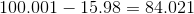Rounding to two decimal places gives us.

← Previous 1 3

### All High School Chemistry Resources